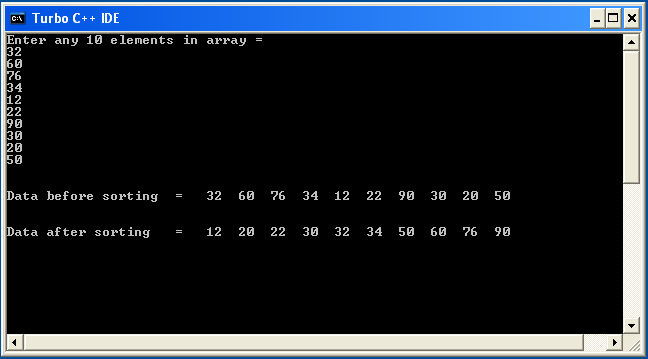# Sort an Array Elements Ascending Order in C

## Sort an Array Elements in Ascending Order Program in C

Sort array elements means arrange elements of array in Ascending Order and Descending Order. we need to sort the given array in ascending order such that elements will be arranged from smallest to largest. This can be achieved through two loops. The outer loop will select an element, and inner loop allows us to compare selected element with rest of the elements.

### Algorithm Sort an Array Elements in Ascending Order

• Declare and initialize an array.
• Loop through the array and select an element.
• The inner loop will be used to compare the selected element from the outer loop with the rest of the elements of the array.
• If any element is less than the selected element then swap the values.
• Continue this process till entire array is sorted in ascending order.

### Different Ways to Sort Array Elements

There are literally hundreds of different ways to sort arrays. Below are the top ways to sort an array element in ascending or descending order.

## Sort an Array Elements in Ascending Orde Program in C

```#include<stdio.h>
#include<conio.h>

void main()
{
int i,a,temp,j;
clrscr();
printf("Enter any 10 num in array = \n");
for(i=0;i<=10;i++)
{
scanf("%d",&a[i]);
}
printf("\n\nData before sorting  = ");
for(j=0;j<10;j++)
{
printf("  %d",a[j]);
}
for(i=0;i<=10;i++)
{
for(j=0;j<=10-i;j++)
{
if(a[j]>a[j+1])
{
temp=a[j];
a[j]=a[j+1];
a[j+1]=temp;
}
}
}
printf("\n\n\nData after sorting   = ");
for(j=0;j<10;j++)
{
printf("  %d", a[j]);
}
getch();
}
```

OutputWIFI CCTV Camera